Anúncio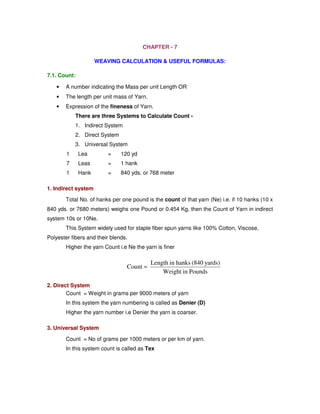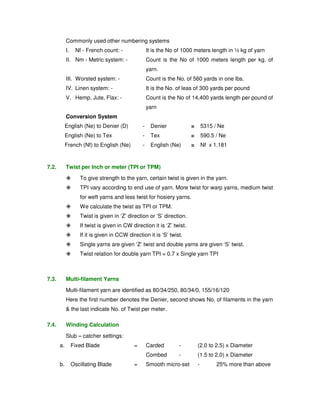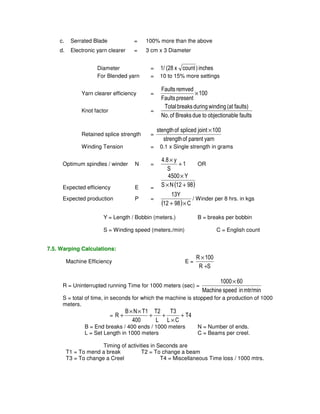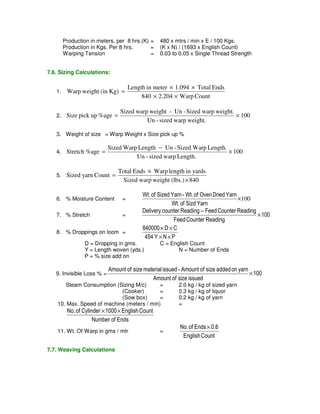Anúncio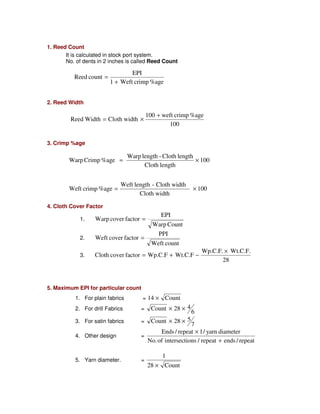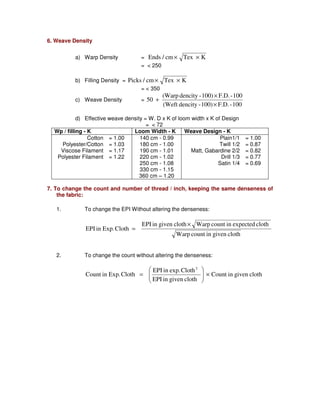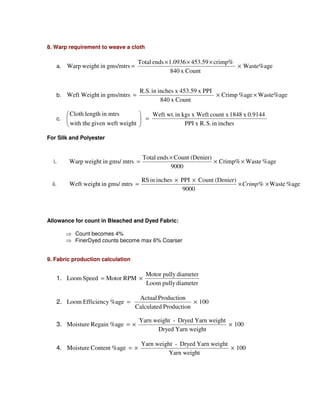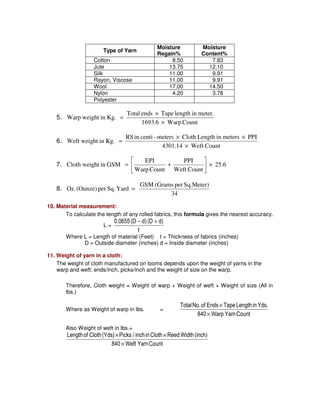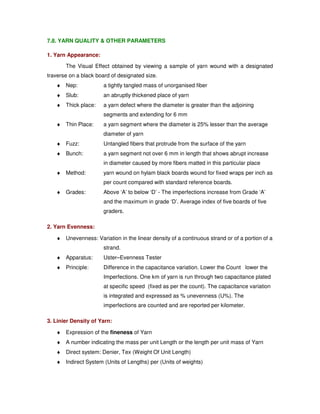Anúncio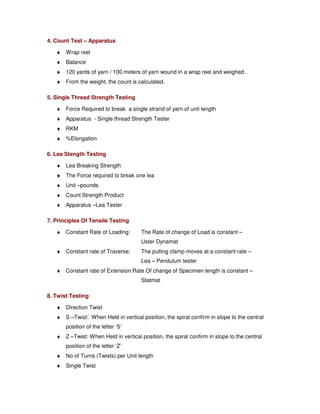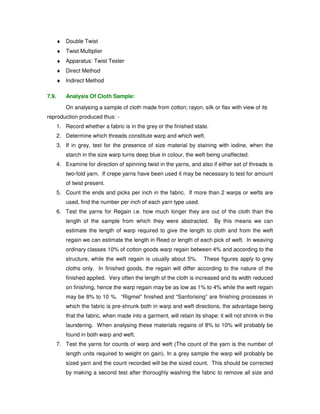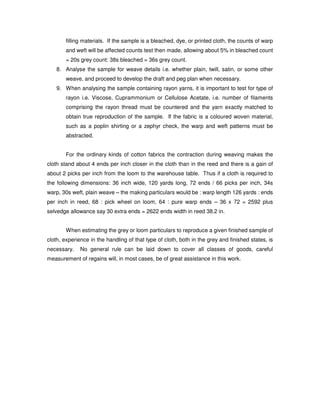Próximos SlideShares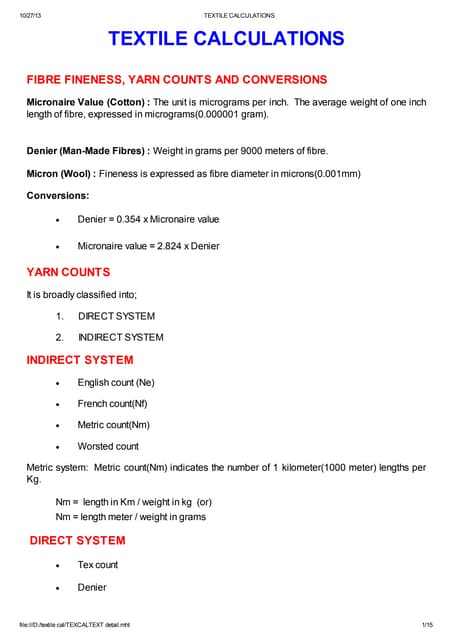Textile calculations
Carregando em ... 3
1 de 12
Anúncio

### Weaving calculation

1. CHAPTER - 7 WEAVING CALCULATION & USEFUL FORMULAS: 7.1. Count: • A number indicating the Mass per unit Length OR • The length per unit mass of Yarn. • Expression of the fineness of Yarn. There are three Systems to Calculate Count - 1. Indirect System 2. Direct System 3. Universal System 1 Lea = 120 yd 7 Leas = 1 hank 1 Hank = 840 yds. or 768 meter 1. Indirect system Total No. of hanks per one pound is the count of that yarn (Ne) i.e. if 10 hanks (10 x 840 yds. or 7680 meters) weighs one Pound or 0.454 Kg, then the Count of Yarn in indirect system 10s or 10Ne. This System widely used for staple fiber spun yarns like 100% Cotton, Viscose, Polyester fibers and their blends. Higher the yarn Count i.e Ne the yarn is finer 2. Direct System Length in hanks (840 yards) Weight in Pounds Count = Count = Weight in grams per 9000 meters of yarn In this system the yarn numbering is called as Denier (D) Higher the yarn number i.e Denier the yarn is coarser. 3. Universal System Count = No of grams per 1000 meters or per km of yarn. In this system count is called as Tex
2. Commonly used other numbering systems I. Nf - French count: - It is the No of 1000 meters length in ½ kg of yarn II. Nm - Metric system: - Count is the No of 1000 meters length per kg. of yarn. III. Worsted system: - Count is the No. of 560 yards in one lbs. IV. Linen system: - It is the No. of leas of 300 yards per pound V. Hemp, Jute, Flax: - Count is the No of 14,400 yards length per pound of yarn Conversion System English (Ne) to Denier (D) - Denier = 5315 / Ne English (Ne) to Tex - Tex = 590.5 / Ne French (Nf) to English (Ne) - English (Ne) = Nf x 1.181 7.2. Twist per Inch or meter (TPI or TPM) To give strength to the yarn, certain twist is given in the yarn. TPI vary according to end use of yarn. More twist for warp yarns, medium twist for weft yarns and less twist for hosiery yarns. We calculate the twist as TPI or TPM. Twist is given in ‘Z’ direction or ‘S’ direction. If twist is given in CW direction it is ‘Z’ twist. If it is given in CCW direction it is ‘S’ twist. Single yarns are given ‘Z’ twist and double yarns are given ‘S’ twist. Twist relation for double yarn TPI = 0.7 x Single yarn TPI 7.3. Multi-filament Yarns Multi-filament yarn are identified as 80/34/250, 80/34/0, 155/16/120 Here the first number denotes the Denier, second shows No. of filaments in the yarn the last indicate No. of Twist per meter. 7.4. Winding Calculation Slub – catcher settings: a. Fixed Blade = Carded - (2.0 to 2.5) x Diameter Combed - (1.5 to 2.0) x Diameter b. Oscillating Blade = Smooth micro-set - 25% more than above
3. c. Serrated Blade = 100% more than the above d. Electronic yarn clearer = 3 cm x 3 Diameter Diameter = 1/ (28 x count ) inches For Blended yarn = 10 to 15% more settings Faults remved Yarn clearer efficiency = 100 Faults present × Knot factor = Total breaks during winding (at faults) No. of Breaks due to objectionable faults Retained splice strength = stength of spliced joint × 100 strength of parent yarn Winding Tension = 0.1 x Single strength in grams 4.8 y Optimum spindles / winder N = 1 S + × OR 4500 × Y × + Expected efficiency E = S N (12 98) Expected production P = 13Y + × (12 98) C / Winder per 8 hrs. in kgs Y = Length / Bobbin (meters.) B = breaks per bobbin S = Winding speed (meters./min) C = English count 7.5. Warping Calculations: Machine Efficiency E = R × 100 R + S R = Uninterrupted running Time for 1000 meters (sec) = 1000× 60 Machine speed in mtr/min S = total of time, in seconds for which the machine is stopped for a production of 1000 meters. T3 T2 B N T1 = R + T4 L C L 400 × + + × × + B = End breaks / 400 ends / 1000 meters N = Number of ends. L = Set Length in 1000 meters C = Beams per creel. Timing of activities in Seconds are T1 = To mend a break T2 = To change a beam T3 = To change a Creel T4 = Miscellaneous Time loss / 1000 mtrs.
4. Production in meters. per 8 hrs.(K) = 480 x mtrs / min x E / 100 Kgs. Production in Kgs. Per 8 hrs. = (K x N) / (1693 x English Count) Warping Tension = 0.03 to 0.05 x Single Thread Strength 7.6. Sizing Calculations: 1. Length in meter × 1.094 × Total Ends 840 2.204 Warp Count Warp weight (in Kg) × × = Sized warp weight - Un -Sized warp weight. 2. Size pick up %age × 100 Un - sized warp weight. = 3. Weight of size = Warp Weight x Size pick up % Sized Warp Length − Un -Sized Warp Length. Stretch %age × 4. 100 Un - sized warp Length. = 5. Total Ends Warp length in yards × × = Sized warp weight (lbs.) 840 Sized yarn Count Wt. of Sized Yarn - Wt. of Oven Dried Yarn 6. % Moisture Content = ×100 Wt. of Sizd Yarn Delivery counter Reading Feed Counter Reading 7. % Stretch = 100 Feed Counter Reading × − 8. % Droppings on loom = 840000 × D × C 454 Y × N × P D = Dropping in gms. C = English Count Y = Length woven (yds.) N = Number of Ends P = % size add on Amount of size material issued - Amount of size added on yarn 9. Invisible Loss % = 100 Amount of size issued × Steam Consumption (Sizing M/c) = 2.0 kg / kg of sized yarn (Cooker) = 0.3 kg / kg of liquor (Sow box) = 0.2 kg / kg of yarn 10. Max. Speed of machine (meters / min) = No. of Cylinder × 1000 ×English Count Number of Ends 11. Wt. Of Warp in gms / mtr = No. of Ends × 0.6 English Count 7.7. Weaving Calculations
5. 1. Reed Count It is calculated in stock port system. No. of dents in 2 inches is called Reed Count 2. Reed Width 3. Crimp %age 100 weft crimp %age 100 Warp Crimp %age = × Weft crimp %age = × 4. Cloth Cover Factor 1. 1 Weft crimp %age Warp length - Cloth length Weft length - Cloth width EPI Warp Count Warp cover factor = 2. PPI Weft count Weft cover factor = 3. Wp.C.F. Wt.C.F. 28 Cloth cover factor Wp.C.F Wt.C.F × = + − 5. Maximum EPI for particular count 1. For plain fabrics = 14 × Count 2. For drill Fabrics = 6 Count × 28 × 4 3. For satin fabrics = 7 Count × 28 × 5 4. Other design = Ends / repeat 1/ yarn diameter No. of intersections / repeat + ends / repeat × 5. Yarn diameter. = 1 28 × Count EPI Reed count + = 100 Cloth length Reed Width Cloth width + = × 100 Cloth width
6. 6. Weave Density a) Warp Density = Ends / cm × Tex × K = 250 b) Filling Density = Picks / cm × Tex × K = 350 c) Weave Density = (Warp dencity -100) × F.D. - 100 (Weft dencity - 100) F.D. - 100 50 × + d) Effective weave density = W. D x K of loom width x K of Design = 72 Wp / filling - K Loom Width - K Weave Design - K Cotton = 1.00 140 cm - 0.99 Plain1/1 = 1.00 Polyester/Cotton = 1.03 180 cm - 1.00 Twill 1/2 = 0.87 Viscose Filament = 1.17 190 cm - 1.01 Matt, Gabardine 2/2 = 0.82 Polyester Filament = 1.22 220 cm - 1.02 Drill 1/3 = 0.77 250 cm - 1.08 Satin 1/4 = 0.69 330 cm - 1.15 360 cm – 1.20 7. To change the count and number of thread / inch, keeping the same denseness of the fabric: 1. To change the EPI Without altering the denseness: EPI in given cloth Warp count in expected cloth Warp count in given cloth EPI in Exp. Cloth × = 2. To change the count without altering the denseness: Count in given cloth EPI in exp. Cloth EPI in given cloth Count in Exp. Cloth 2 × =
7. 8. Warp requirement to weave a cloth Total ends × 1.0936 × 453.59 × crimp% a. Warp weight in gms/mtrs × Waste%age 840 x Count = R.S. in inches x 453.59 x PPI Weft Weight in gms/mtrs = × × b. Crimp %age Waste%age 840 x Count c. Weft wt. in kgs x Weft count x 1848 x 0.9144 PPI x R. S. in inches Cloth length in mtrs with the given weft weight = For Silk and Polyester Total ends × Count (Denier) i. Warp weight in gms/ mtrs × Crimp% × Waste %age 9000 = RS in inches × PPI × Count (Denier) ii. Weft weight in gms/ mtrs = × Crimp % × Waste %age 9000 Allowance for count in Bleached and Dyed Fabric: Count becomes 4% FinerDyed counts become max 6% Coarser 9. Fabric production calculation 1. Motor pully diameter Loom pully diameter Loom Speed = Motor RPM × Actual Production 2. Loom Efficiency %age = × 100 Calculated Production Yarn weight - Dryed Yarn weight 3. Moisture Regain %age = × × 100 Dryed Yarn weight Yarn weight - Dryed Yarn weight 4. Moisture Content %age = × × 100 Yarn weight
8. Type of Yarn Moisture Regain% Moisture Content% Cotton 8.50 7.83 Jute 13.75 12.10 Silk 11.00 9.91 Rayon, Viscose 11.00 9.91 Wool 17.00 14.50 Nylon 4.20 3.78 Polyester 5. Total ends Tape length in meter. 1693.6 Warp Count Warp weight in Kg. × × = 6. RS in centi -meters × Cloth Length in meters × PPI 4301.14 Weft Count Weft weight in Kg. × = PPI EPI 7. 25.6 Cloth weig ht in GSM = + Warp Count Weft Count ×
9. 8. GSM (Grams per Sq.Meter) 34 Oz. (Ounze) per Sq. Yard = 10. Material measurement: To calculate the length of any rolled fabrics, this formula gives the nearest accuracy. L = 0.0655 (D − d) (D + d) t Where L = Length of material (Feet) t = Thickness of fabrics (inches) D = Outside diameter (inches) d = Inside diameter (inches) 11. Weight of yarn in a cloth: The weight of cloth manufactured on looms depends upon the weight of yarns in the warp and weft: ends/inch, picks/inch and the weight of size on the warp. Therefore, Cloth weight = Weight of warp + Weight of weft + Weight of size (All in lbs.) Where as Weight of warp in lbs. = Total No. of Ends Tape Length in Yds. 840 × Warp Yarn Count × Also Weight of weft in lbs.= Length of Cloth (Yds) × Picks / inch in Cloth × Reed Width (inch) 840 × Weft Yarn Count
10. 7.8. YARN QUALITY OTHER PARAMETERS 1. Yarn Appearance: The Visual Effect obtained by viewing a sample of yarn wound with a designated traverse on a black board of designated size. ¨ Nep: a tightly tangled mass of unorganised fiber ¨ Slub: an abruptly thickened place of yarn ¨ Thick place: a yarn defect where the diameter is greater than the adjoining segments and extending for 6 mm ¨ Thin Place: a yarn segment where the diameter is 25% lesser than the average diameter of yarn ¨ Fuzz: Untangled fibers that protrude from the surface of the yarn ¨ Bunch: a yarn segment not over 6 mm in length that shows abrupt increase in diameter caused by more fibers matted in this particular place ¨ Method: yarn wound on hylam black boards wound for fixed wraps per inch as per count compared with standard reference boards. ¨ Grades: Above ‘A’ to below ‘D’ - The imperfections increase from Grade ‘A’ and the maximum in grade ‘D’. Average index of five boards of five graders. 2. Yarn Evenness: ¨ Unevenness: Variation in the linear density of a continuous strand or of a portion of a strand. ¨ Apparatus: Uster–Evenness Tester ¨ Principle: Difference in the capacitance variation. Lower the Count lower the Imperfections. One km of yarn is run through two capacitance plated at specific speed (fixed as per the count). The capacitance variation is integrated and expressed as % unevenness (U%). The imperfections are counted and are reported per kilometer. 3. Linier Density of Yarn: ¨ Expression of the fineness of Yarn ¨ A number indicating the mass per unit Length or the length per unit mass of Yarn ¨ Direct system: Denier, Tex (Weight Of Unit Length) ¨ Indirect System (Units of Lengths) per (Units of weights)
11. 4. Count Test – Apparatus ¨ Wrap reel ¨ Balance ¨ 120 yards of yarn / 100 meters of yarn wound in a wrap reel and weighed . ¨ From the weight, the count is calculated. 5. Single Thread Strength Testing ¨ Force Required to break a single strand of yarn of unit length ¨ Apparatus - Single thread Strength Tester ¨ RKM ¨ %Elongation 6. Lea Stength Testing ¨ Lea Breaking Strength ¨ The Force required to break one lea ¨ Unit –pounds ¨ Count Strength Product ¨ Apparatus –Lea Tester 7. Principles Of Tensile Testing ¨ Constant Rate of Loading: The Rate of change of Load is constant – Uster Dynamat ¨ Constant rate of Traverse: The pulling clamp moves at a constant rate – Lea – Pendulum tester ¨ Constant rate of Extension:Rate Of change of Specimen length is constant – Statmat 8. Twist Testing ¨ Direction Twist ¨ S –Twist: When Held in vertical position, the spiral confirm in slope to the central position of the letter ‘S’ ¨ Z –Twist: When Held in vertical position, the spiral confirm in slope to the central position of the letter ‘Z’ ¨ No of Turns (Twists) per Unit length ¨ Single Twist
12. ¨ Double Twist ¨ Twist Multiplier ¨ Apparatus: Twist Tester ¨ Direct Method ¨ Indirect Method 7.9. Analysis Of Cloth Sample: On analysing a sample of cloth made from cotton; rayon, silk or flax with view of its reproduction produced thus: - 1. Record whether a fabric is in the grey or the finished state. 2. Determine which threads constitute warp and which weft. 3. If in grey, test for the presence of size material by staining with iodine, when the starch in the size warp turns deep blue in colour, the weft being unaffected. 4. Examine for direction of spinning twist in the yarns, and also if either set of threads is two-fold yarn. If crepe yarns have been used it may be necessary to test for amount of twist present. 5. Count the ends and picks per inch in the fabric. If more than 2 warps or wefts are used, find the number per inch of each yarn type used. 6. Test the yarns for Regain i.e. how much longer they are out of the cloth than the length of the sample from which they were abstracted. By this means we can estimate the length of warp required to give the length to cloth and from the weft regain we can estimate the length in Reed or length of each pick of weft. In weaving ordinary classes 10% of cotton goods warp regain between 4% and according to the structure, while the weft regain is usually about 5%. These figures apply to grey cloths only. In finished goods, the regain will differ according to the nature of the finished applied. Very often the length of the cloth is increased and its width reduced on finishing, hence the warp regain may be as low as 1% to 4% while the weft regain may be 8% to 10 %. “Rigmel” finished and “Sanforising” are finishing processes in which the fabric is pre-shrunk both in warp and weft directions, the advantage being that the fabric, when made into a garment, will retain its shape: it will not shrink in the laundering. When analysing these materials regains of 8% to 10% will probably be found in both warp and weft. 7. Test the yarns for counts of warp and weft (The count of the yarn is the number of length units required to weight on gain). In a grey sample the warp will probably be sized yarn and the count recorded will be the sized count. This should be corrected by making a second test after thoroughly washing the fabric to remove all size and
13. filling materials. If the sample is a bleached, dye, or printed cloth, the counts of warp and weft will be affected counts test then made, allowing about 5% in bleached count = 20s grey count: 38s bleached = 36s grey count. 8. Analyse the sample for weave details i.e. whether plain, twill, satin, or some other weave, and proceed to develop the draft and peg plan when necessary. 9. When analysing the sample containing rayon yarns, it is important to test for type of rayon i.e. Viscose, Cuprammonium or Cellulose Acetate, i.e. number of filaments comprising the rayon thread must be countered and the yarn exactly matched to obtain true reproduction of the sample. If the fabric is a coloured woven material, such as a poplin shirting or a zephyr check, the warp and weft patterns must be abstracted. For the ordinary kinds of cotton fabrics the contraction during weaving makes the cloth stand about 4 ends per inch closer in the cloth than in the reed and there is a gain of about 2 picks per inch from the loom to the warehouse table. Thus if a cloth is required to the following dimensions: 36 inch wide, 120 yards long, 72 ends / 66 picks per inch, 34s warp, 30s weft, plain weave – the making particulars would be : warp length 126 yards : ends per inch in reed, 68 : pick wheel on loom, 64 : pure warp ends – 36 x 72 = 2592 plus selvedge allowance say 30 extra ends = 2622 ends width in reed 38.2 in. When estimating the grey or loom particulars to reproduce a given finished sample of cloth, experience in the handling of that type of cloth, both in the grey and finished states, is necessary. No general rule can be laid down to cover all classes of goods, careful measurement of regains will, in most cases, be of great assistance in this work.
Anúncio